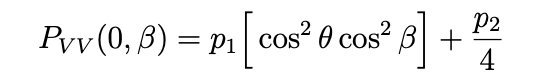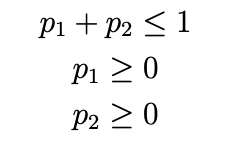# Fixing the value of the sum of multiple parameters

Hi,
I was wondering if there is a way to fix parameter limits on the sum of multiple parameters. Something like:
`g->FixPar(1+2+3,1)`
where 1,2,3 are fitting parameters and 1 is the value to fix the sum to. Is this in any way possible? I am trying to implement a constraint, which is why this would be useful.

_ROOT Version: 6.23/01
Platform: MacBook Pro
Compiler: Not Provided

Perhaps @moneta can help?

Hi,

This is currently not possible. There is not yet support for constraints which consists of function of parameters, instead only simple parameter bounds are supported.
In some simple cases as above, can be re-formulated by some parameter transformation .
For example defining `q1 = p1; q2 = p2; q3 = p1+p2+p3; `

Lorenzo

1 Like

Ah ok, I think this would work, thank you moneta!

Hello again,
It makes sense when you explain it, but I have trouble implementing it. Do you know how I could implement the following?where.
Like how would I code the above equation as a TF1 pointer? Can you help?
The function is a function of only beta, and it has three parameters, p_1, theta, and p_2.